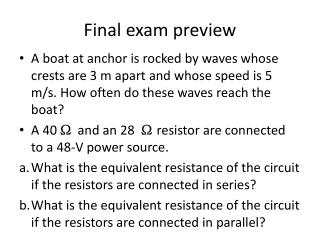DownloadDownload PresentationFinal exam preview

# Final exam preview

Télécharger la présentation## Final exam preview

- - - - - - - - - - - - - - - - - - - - - - - - - - - E N D - - - - - - - - - - - - - - - - - - - - - - - - - - -
##### Presentation Transcript

1. Final exam preview • A boat at anchor is rocked by waves whose crests are 3 m apart and whose speed is 5 m/s. How often do these waves reach the boat? • A 40 Ωand an 28 Ωresistor are connected to a 48-V power source. a. What is the equivalent resistance of the circuit if the resistors are connected in series? b. What is the equivalent resistance of the circuit if the resistors are connected in parallel?

2. Final exam preview • A microwave draws a 7.5-A current from a 120-V power source. a. How much power is delivered to the microwave? b. How much energy does the microwave use to heat food for 3 minutes?

3. Final exam preview • What is the force between two small charged spheres that have charges of 4 x10–7C and 7x10–7C and are placed 90 cm apart in air? • What is the value of charge of a body that carries 250 excess electrons?

4. Final exam preview • In a television picture tube, the work done in moving an electron is 50 x10–18J. What is the potential difference inside the picture tube? • What is the quantity of charge that passes through a conductor when there is a current of 0.65 A for 20 minutes?

5. Final exam preview • Sphere A, with a charge of +12.0 µC, is placed at a distance of 5.0 cm from another sphere B. The charge on sphere B is –7.0 µC and is located to the right of sphere A. A third sphere C is placed 8.0 cm beneath sphere A. The charge on sphere C is +6.0 µC. Determine the net force on sphere A.

6. Final exam preview • A positive charge of 6 μC is located in an electric field of intensity 5.00 N/C directed toward the south. Find the magnitude and the direction of the force on the test charge. • A battery of potential difference 12 V delivers 25 J to a conductor. What is the quantity of charge that flows through the conductor?

7. Final exam preview • A current of 2.0 A flows for 15.0 minutes through a bulb and delivers 8500 J of electric energy to the bulb. What is the potential difference across the bulb? • A 12-V potential difference is applied across a series combination of a 6-ohm resistor and a 45-ohm resistor. What is the current in the 45-ohm resistor?

8. Final exam preview • How much heat must be removed from 5 kg of water to cool it from 90°C to 5°C? The specific heat capacity of water is 4182 J/kg K. • A pendulum on a different planet with a length of 3 m has a period of 2 s. What is the acceleration due to gravity at the pendulum’s location?

9. Final exam preview • A battery does 12000 J of work on 48 coulombs of charge. What voltage does the battery supply? • A certain bulb with a resistance of 130 ohms is labeled 443 W. For what voltage circuit was this bulb designed?

10. Final exam preview • A lifeguard on a beach observes that waves have a speed of 7 m/s and a distance of 1.5 m between wave crests. What is the period of the wave motion? • A positive test charge of 6.00 μC is placed in an electric field, which exerts a force of 5.00x 10–4N on the test charge. What is the magnitude of the electric field strength at the location of the test charge?

11. Final exam preview • How much energy is consumed in 35 minutes by a bulb with a resistance of 28 W when connected to a 12-V battery? • What is the equivalent resistance of a 36-ohm and a 60-ohm resistor connected in parallel?

12. Final exam preview • The sound a mosquito makes is produced when it beats its wings at the average frequency of 620 wing beats per second. What is the wavelength of the sound waves produced by the mosquito? Assume V = 340

13. Final exam preview • An electron traveling 5x 107m/s at a right angle to a magnetic field experiences a force of 8 x 10–13N. What is the strength of the magnetic field? • An object of height 8.0 cm is placed at a distance of 7 cm from a convex lens of focal length 4.0 cm. a. Find the position of the image.

14. Final exam preview • A 36-V potential difference is applied across a parallel combination of a 80-ohm and a 20-ohm resistor. What is the current in the 20-ohm resistor? • What is the current in a 75-W bulb connected to a 110-V source?

15. Final exam preview • Sonya hears water dripping from the eaves of the house onto a porch roof. She counts 125 drops in 2.0 min. a. What is the frequency of the drops? b. What is the period of the drops?

16. Final exam preview • What is the magnitude of the force on an electron in an electric field of 48 N/C? • Waves in a lake are 2 m in length and pass an anchored boat 1.5 s apart. What is the speed of the waves?